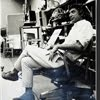## Eugene Ray SDSU 1970'sEugene Ray 1970's. photo credit: Tom Davis

## Sunday, November 18, 2012

### PICTORS METAMORPHISIS was a remodel done in 1976 w/ aid of= STUDENTS, COOKS & BUSBOYS ! ^^^^^^^^^^^^^^^^^^^^^ "SUPERGRAPHIC" SIGNAGE was with philodendrin selloum my > >TRANSCENDENTAL ALCHEMY< ^^^^^^^^^^^^^^^^^^^^ LOW BUDGET GIFT to the public was my practice @ sdsu for years ^^^^^^^^^^^^^^^^^^^^^ 5 PHOTOS REVEAL OUR WORK > radiant color signs were a way> to a tropic/ affordable solution- ( >painted in my spare time< ) !+PICTORS METAMORPHISIS+
^^^^^^^^^^^^^^^
>for five years at the beginning of a<
>quarter century at sdsu i was able<
>to construct several projects<
+"GRATIS"+
>for the public who needed help<
^^^^^^^^^^^^^^^^^
>then i was told if a student<
>was hurt it was my fault<
^^^^^^^^^^^^
+THAT CURTAILED MY EFFORT+
(+BUT ONLY PARTIALLY+)
^^^^^^^^^^^
>pictors was a low budget remodel<
>project for two cooks who came<
+SEEKING DESIGN HELP+
^^^^^^^^^^^^
>reminds me of the jazz workshop<
>in new orleans when two<
+MUSICIANS+
+SOUGHT DESIGN HELP+
^^^^^^^^^^^^^

(>magic matrix alchemy<
>of a low/no budget<)
^^^^^^^^
+F O R M U L A+
>using martin senour paints w/ a<
>brillient european pigment and<
+TROPICAL MATRIX+
>a tropical planter out in front<
^^^^^^^^^^^^^^^^^
+TRANSCENDENTAL+
+MANIFESTATION+
^^^^^^^^^
>suddenly appeared on india street<
>an old san diego out of the way<
+MISE-EN-SCENE+
^^^^^^^^^
+MIRACLE HAPPENED+
>(very much like a crop circle)<
^^^^^^^^^^^^

+TONIGHT'S PHOTOS+
>convey the manifest on old<
>india street in san diego<
^^^^^^^^^^^^
>here to match the text, thanks<)
^^^^^^^^^^^^^^

#1)          +PICTORS 1976+
>the finished project with tropical<
>plants & signage all in place<
^^^^^^^^^^^^^^^
(>unfortunately i have not<
>found a "before" photo<)
^^^^^^^^^^^^^

#2)          +TWO CONCEPTS+
>i designed two versions of the<
(>graphite on vellum<)
^^^^^^^^^^^
+TOP ONE SELECTED+
(>symbols in circles<
>exotic produce<)
^^^^^^^

#3)      +RAISING CEREMONY+
>the cooks, busboys and a few of our<
>students perform the raising of<
+SPECIAL MOMENT+
^^^^^^^^^^^^^^

>two inch redwood "bender board"<
>strips on a metal frame created<
^^^^^^^^^^^^^^^

#5)      +TROPICAL SIGNAGE+
>reflected the tropical cusine offered
>in this very exotic restaurant and<
(+AFRICAN CUISINE+)
>light fixtures inside provided<
>more of this theme flavor<
^^^^^^^^^^^^^^

+TROPIQUE-MAGIQUE+
+METAPHYSIQUE+
^^^^^^^^
>san diego was connected to exotic<
>places once again, as it had been<
^^^^^^^^^^^^^^^^^^^
>(attention mr james bourke)<
+IN ITS EARLY SAIL DAYS+
^^^^^^^^^^^^
gene ray, lajolla, california

>eugene ray. mfa, architect<
>professor emeritus, sdsu<
^^^^^^^^^^^^^